Electromechanical Indicating Type Instruments - 3

# Electromechanical Indicating Type Instruments - 3 - Electrical Engineering (EE)

 1 Crore+ students have signed up on EduRev. Have you?

Moving Iron Type Instrument:
• The most common Ammeters and Voltmeters for laboratory or switch board used at power frequencies are the Moving iron instruments.
• M. I. type instrument may be of attraction type moving iron or repulsion type moving iron.
⇒ Attraction type M. I. instrument is shown below in figure.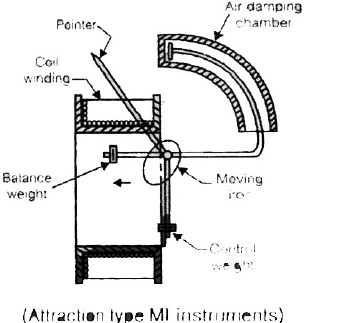• Attraction type consist of movable iron vane attached to the spindle. Iron vane is always attracted towards the centre of fixed coil.
• When the current flows through the coil, a magnetic field is produced and MI moves from weaker field outside the coil to the stronger field inside it or in other words the MI is attracted in.
• The controlling torque (Tc) is provided by springs but gravity control can be used for pannel of instruments.
• Damping is provided by “Air-friction damping” with the help of “Light Al-piston” which moves in a fixed chamber closed at one end as shown in above figure
• The operating magnetic field in MI is very weak so, eddy current damping in not used.
• Range of magnetic field is 0.0062 to 0.0075 wb/ m2.
• If frequency of input is large the eddy current induced in iron vane may distort the main flux or field of the coil. Therefore, frequency of signal in moving iron type instrument is restricted to 0 - 125 Hz.

Note:
⇒ In both type (Attraction or repulsion) of MI instrument, the iron vanes are magnetised in such a way by the current that there is always a force of attraction in the “Attraction type” and repulsion in the “Repulsion type”. Thus we c an say Moving iron type instrument are unpolarised instruments i. e. They are independent of the direction in which the current flows.

So, these “MI instruments” can be used on both AC and DC purposes.

Expression for deflecting torque in MI type: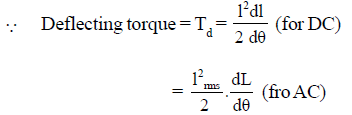where,
L = inductance of coil.
I = current through the coil.
θ = deflection of the pointer.
And control torque,
Tc = k θ
At balance condition or at final deflectional
T= Td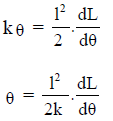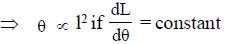⇒ The scale is non-linear or non-uniform because θ ∝ I2

Note:
⇒ Moving iron type instrument measured RMS value of input quantity.
⇒ MI type instruments are less accurate but cheaper.

Electrodynamometer Type instrument: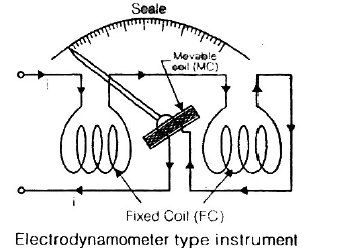• Electordynamometer type instruments consists of two coil, fixed ciol (FC) and Moving ciol (MC).
• Fixed coil is splitted into two part in order to have uniform distribution of flux around moving ciol.
• FC is used to provide operating field.
• MC is a current carrying coil on which deflection torque (Td) is produced.
• For measurement of voltage and current both coils are connected inseeries. Deflection produced in moving coil is same for a.c. and d.c. and is proportional to the r.m.s.value of a.c. Therefore, these are first calibrated on d.c. This calibration is then transferred to the a.c. instrument on alternating current.
• Spring control is used and Control spring is provided for dual purposes as,

(i) To generate spring control torque.
(ii) Provide path for current to the moving coil.

• If spring is broken, controlling torque is zero and Meter reads zero.
• Air-friction damping is used here.

Single-phase Electrodynamometer Power Factor Meter:

• he power factor, by definition is the cosine of the phase angle between voltage and current, and power-factor measurements usually involve the determination of this phase angle.
• The construction of a single-phase electrodynamometer power factor meter is shown in figure below.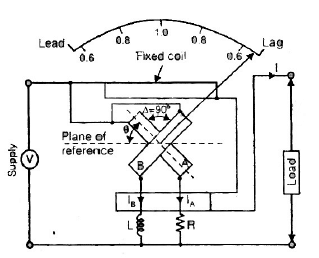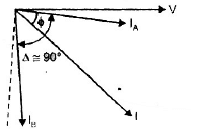.

• This is demonstrated in the operation of the crossed-coil power-factor meter. In this instruments. the moving element consists of two coil, mounted on the same shaft but at right angles to each other. The moving coils rotate in the magnetic field provided by the field coil that carries the line current.
• One coil of the movable element is connected in series with a resistor across the lines and receives its current from the applied potential difference.
• The second coil of the movable element is connected in series with an inductor across the lines. Since no-control springs are used, the balance position of the movable element depends on the resulting torque developed by the two crossed coils.
• When the movable element is in a balance position, the contribution to the total torque by each element must be equal but of opposite sign.
• The developed torque in each coil is a function of the current through the coil and therefore depends on the impedance of the coil circuit.
• The torque is also proportional to the mutual inductance between each part of the crossed coil and the stationary field coil.
• This mutual inductance depends on the angular position of the crossed-coil elements with respect to the position of the stationary fields coil.
• When the movable element is at balance it can be shown that its angular displacement is a function of the phase-angle between line current
• (field coil) and line voltage (crossed coils). The indication of the pointer, which is connected to the movable element is calibrated directly in terms of the phase angle or power factor.

⇒Let us consider the case of a lagging power factor of cosø .
Deflecting torque acting on coil A is,

TA = KVI Mmax cosø sinθ
θ = angular deflection from the plane of reference
Mmax = Maximum value of mutual inductance between the two coils.
This towque say acts in th eclock-wise direction.

Direction torque acting on coil B is;

lB = KVI Mmax cos (90º – ø )sin (90º + θ ) = KVI Mmax sinø cosθ

This torque acts in the anti-clockwise direction.
Hence at equilibrium,
T= TB

or KVI Mmax cosø sinθ = KVI Mmax sinø cosθ

cosø sinθ = sinø cosθ

cosø sinθ - sinø cosθ = 0

sin(ø - θ) = 0

(ø - θ) = 0

ø = θ

Watthour Meter :
• It is not often found in a laboratory but it is widely used for the commercial measurement of electrical energy.
• Figure below shows the elements of a single phase watthour meter.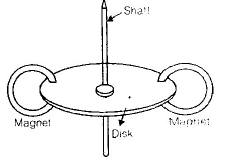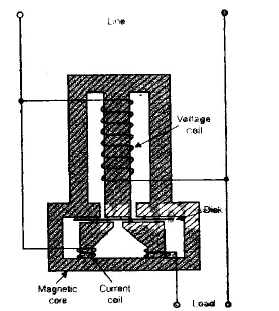• In the figure above, both coils (current coils and voltage coils) are would on a metal frame of special design, poviding two magnetic circuits.
• A light Al-disk is suspended in the air gap of current-coil field, which causes eddly currents to flow in the disk.
• The developed torque is proportional to the Fieldstrength of the voltage coils and eddly currents in the disk. The no. of rotations of the disk is therefore proportional to the energy consumed by the load in a curtain time internal, and is measured in terms of kilowatt hours (kWh).
• Damping of the disk is provided by the two small permanent magnets located opposite each other at the rim of disk.

Instrument Transformers :
Instrument transformers are employed for ac measurements (current, voltage, power and energy) in conjunction with the relevant instruments. Such transformers are of two types- Current (or series) transformer and potential (or parallel) transformers.

Current transformer is employed whenever the current of an ac circuit exceeds the sage current of the measuring instrument (e.g. ammeter or wattmeter or energy meter).

Potential transformer is employed whenever and wherever the voltage of an ac circuit exceeds the voltage of 750 volts as it is not easy to provide adequate insulation on measuring instruments for voltage above 750 volts.

(a) Current Transformers (CTs)
The Primary of the CT, which consists of only one of a few turns, is connected in series with the load circuit and so carries the load current to be measured. The secondary winding consists of many more turns than the primary and have the instrument ( an ammeter or ammeter and current coil of wattmeter) directly connected across it. In case of current transformer as the load impedance or 'burden' on the secondary is very small, so the current transformer operates on short-circuit conditions. Also the current in secondary winding is not governed by the load impedance on the secondary but depends upon the current flowing in the primary winding (i.e. main circuit current.) The amount of power which the CT handles is small. The product of voltage and current on the secondary side when the CT is supplying the instrument with its maximum rated value of current, is known as rated burden and is measured in volt-amperes (VA).

There are two types of errors introduced by CT into a circuit These are

(i) ratio error (ii) phase angel error.
CT ratio and phase angle errors are given by equations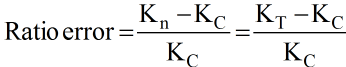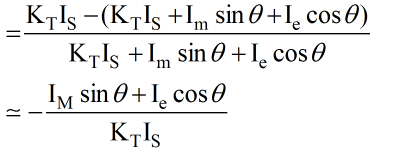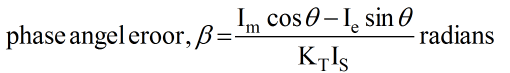Ratio error is taken as + ve when the actual ratio of current transformer is less than nominal ratio. In the above equation of ratio error-ve sign indicates that actual ratio of CT is more than nominal ratio.

The ratio error determines the amount of turns compensation to reduce the error to minimum.

(b) Potential Transformers (PTs)
The potential transformers are used for measurement of high voltages by means of low range voltmeter or for energising the potential coils of wattmeters and energy meters. These are also used for energising relays and other protective equipment. The voltage being measured acts cross the primary winding, which has a large number of turns and connected across the circuit. the secondary winding which has a much smaller number of turns is coupled magnetically through the magnetic circuit to the primary winding. The turn ratio is so adjusted that secondary voltage is applied to the primary.

The errors introduced by PT are less pronounced than those introduced by CTs.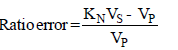where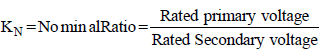Phase angle error,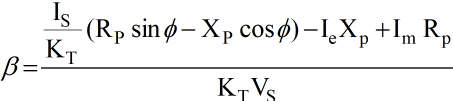The ratio error can be compensated by increasing the turns on secondary winding by the appropriate small amount.

• Instrument transformers (IT) are used to measure AC at generating stations, transformer stations and at transmission lines, in conjunction with AC measuring instruments (Voltmeters, Ammeters, Wattmeters, VARmeters etc.)
• IT performs two important functions and they are :
⇒ They serve to “extend the range” of AC measuring instrument, muxh as the shunt or the multiplier extends the range of DC meter.
⇒ Also, they serve to “isolate” the measuring instrument from the HV power line.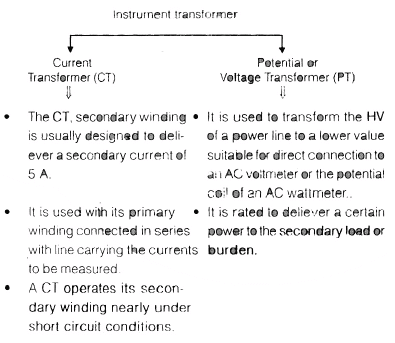The document Electromechanical Indicating Type Instruments - 3 - Electrical Engineering (EE) is a part of Electrical Engineering (EE) category.
All you need of Electrical Engineering (EE) at this link: Electrical Engineering (EE)

Track your progress, build streaks, highlight & save important lessons and more!(Scan QR code)

,

,

,

,

,

,

,

,

,

,

,

,

,

,

,

,

,

,

,

,

,

;